# Varistor

## Varistor

Varistor also known as Voltage Dependent Resistor (VDR) is a passive two-terminal solid state semiconductor device that is used to provide protection to electrical and electronic circuits from overcurrent situations.

The word “Varistor” is a combination of the words VARI-able resi-STOR. Hence, the resistance of a varistor is variable and depends on the voltage applied.

Their resistance decreases when the voltage increases. In case of excessive voltage increases, their resistance drops dramatically. This behavior makes them suitable to protect circuits during voltage surges such as lightning strikes and electrostatic discharges.Fig.1: Varistor

Fig.1 shows a real-life varistor, which may be confused with a capacitor. However, they don’t have anything in common than their size and design.

A Varistor is used to suppress voltage, whereas a capacitor cannot perform such functions.

Nowadays the resistive body of a varistor is made from semiconductor material making it a type of semiconductor resistor with a non-ohmic symmetrical voltage and current characteristics suitable for both AC and DC voltage applications.

### Varistor Symbol

The two standard symbols of varistor are shown below.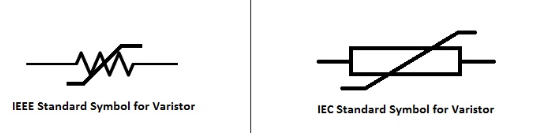### AC Waveform Transients

When a high voltage surge is applied to a circuit, the outcome is usually disastrous to it, therefore the varistor plays an important role in the protection of delicate electronic circuits from switching spikes and overvoltage transients.

Transient surges originate from a variety of electrical circuits and sources regardless of whether they operate from an AC or DC supply as they are often generated within the circuit itself or transmitted into the circuit from external sources.

Transients within a circuit can rise rapidly increasing the voltage to several thousand volts, and it is these voltage spikes which must be prevented from appearing across delicate electronic circuits and components.

One of the most common sources of voltage transients is the L(di/dt) effect caused by the switching of inductive coils and transformer magnetizing currents, DC motor switching applications and surges from the switching-on of fluorescent lighting circuits or other supply surges.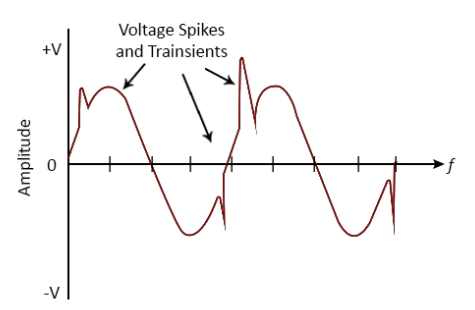Fig.2: AC Transient Waveform of Varistor

The Fig.2 above shows the waveform of an AC transient.

Varistors are connected in circuits across a mains supply either phase-to-neutral, phase-to-phase for AC operation, or positive-to-negative for DC operation and have a voltage rating to suit their application.

A varistor can also be used for DC voltage stabilization and especially for electronic circuit protection against overvoltage pulses.

### Static Resistance of a Varistor

Under normal operating conditions the resistance of a varistor is very high. Thus operating in a similar way to the zener diode by allowing lower threshold voltages to pass unaffected.

However, when the voltage across the varistor (either polarity) exceeds the varistors rated value, its effective resistance falls drastically and continue to decrease with the increase in voltage.

The relation between the static resistance of the varistor and the voltage across it is shown in the figure below.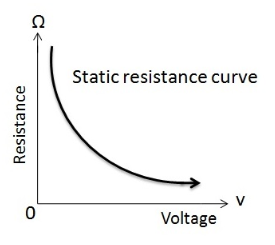Fig.3: Static Resistance VS Voltage

### V-I Characteristics of Varistor

We already know from Ohm’s Law that the current-voltage (I-V) characteristics of a resistor is a straight line provided that R is kept constant. That means the current is directly proportional to the potential difference across the ends of the resistor.

However, in case of a varistor, the I-V curve is not a straight line since a small change of voltage causes a significant change of current (because of the unusual resistance behavior of the varistor).

A typical normalized voltage versus current characteristics curve for a standard varistor is shown below.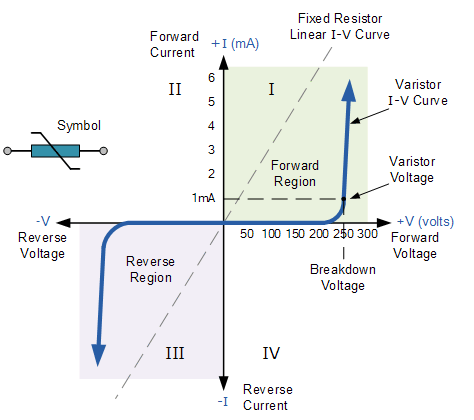Fig.4: V-I Characteristics of Varistor

We can see from the above V-I curve, that a varistor has symmetrical bi-directional characteristics. Which means that it can operate in both directions of a sinusoidal waveform behaving in a similar way to two zener diodes connected back-to-back.

When the varistor is not conducting, the I-V curve shows a linear relationship as the current flowing through the varistor remains constant and low at only a few micro-amperes of “leakage” current.

This is due to its high resistance of the varistor which acts as an open circuit and remains constant until the voltage across the varistor (either polarity) reaches a particular “rated voltage”.

This rated voltage (also known as clamping voltage) is the voltage across the varistor measured with the specified DC current of 1mA.

That is, the DC voltage level applied across its terminals that allows a current of 1mA to flow through the varistors resistive body which itself is dependant upon the materials used in its construction. At this voltage level, the varistor begins to change from its insulating state into its conducting state.

When the transient voltage across the varistor is equal to or greater than the rated value, the resistance of the device suddenly becomes very small turning the varistor into a conductor due to the avalanche effect of its semiconductor material.

The small leakage current flowing through the varistor rapidly rises but the voltage across it is limited to a level just above the varistor voltage.

In other words, the varistor self-regulates the transient voltage across it by allowing more current to flow through it and because of its steep non-linear I-V curve it can pass widely varying currents over a narrow voltage range clipping-off any voltage spikes.

### Capacitance in Varistor

When the applied voltage across the varistor is less than the rated or clamping voltage, a varistor acts as a capacitor rather than a resistor.

This is due to the fact that the main conducting region of a varistor between its two terminals behaves like a dielectric. The two terminals and the dielectric form a capacitor. And this is valid until the voltage reaches the clamping voltage.

Every semiconductor varistor has a capacitance value that depends directly on its area and varies inversely with its thickness.

When used in DC circuits, the capacitance of the varistor remains more or less constant provided that the applied voltage does not increase above the clamping voltage level, and drops off abruptly near towards its maximum rated continuous DC voltage.

However, in AC circuits, this capacitance can affect the body resistance of the device in the non-conducting leakage region of its I-V characteristics. As they are normally connected in parallel with an electric device to protect it against overvoltages, the varistors leakage resistance drops rapidly with an increase in frequency.

The relation between the frequency and the resulting parallel resistance is approximately linear.

The AC reactance, Xc can be calculated using the formula:

Xc  =  1/(2πƒC)

where f= frequency of the circuit

C= Capacitance

Hence as the frequency increases, the leakage current also increases.

### Types of Varistors

The type of Varistors depends on the material type of its body. The two most common types of Varistors are

1. Silicon Carbide Varistor
2. Metal oxide Varistors (MOV)

#### Silicon Carbide Varistor

The body of this type of varistors is made from Silicon Carbide (SiC). It was extensively used once upon a time before the new MOV came to the market. Now they are used intensively in high power, high voltage applications. However, they draw a significant standby current and this is the major drawback of this type of Varistor. Due to this, a series gap is required to limit the stand-by power consumption.

#### Metal oxide Varistors (MOV)

Due to some serious drawbacks of SiC varistors, another type of varistors named metal oxide varistors was developed.

The Metal Oxide Varistor or MOV, is a voltage dependant resistor in which the resistance material is a metallic oxide, primarily zinc oxide (ZnO) pressed into a ceramic like material.

The mixture consists 90 % of Zinc Oxide grains and the other 10 % is made with, other metal oxides like cobalt, bismuth and manganese.

This mixture is sandwiched between two electrodes (metal plates). The filler material acts as a binding agent to zinc oxide grains so that the component is kept intact between two metal plates. The connecting leads of a metal oxide varistor are radial leads.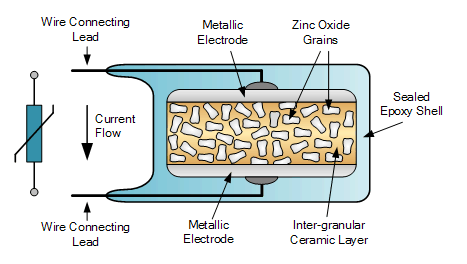Fig.5: Metal Oxide Varistor

Metal oxide varistors are now the most common type of voltage clamping device and are available for use at a wide range of voltages and currents.

The use of a metallic oxide within their construction means that MOV’s are extremely effective in absorbing short term voltage transients and have higher energy handling capabilities.

As with the normal varistor, the metal oxide varistor starts conduction at a specific voltage and stops conduction when the voltage falls below a threshold voltage.

The main differences between a standard silicon carbide (SiC) varistor and a MOV type varistor is that the leakage current through the MOV’s zinc oxide material is very small current at normal operating conditions and its speed of operation in clamping transients is much faster.

MOV’s generally have radial leads and a hard outer blue or black epoxy coating which closely resembles disc ceramic capacitors and can be physically mounted on circuit boards and PCB’s in a similar manner.

### Varistor Specifications

The specifications of a typical varistor are :

Maximum working voltage: It is the peak steady state DC voltage or sinusoidal rms voltage that can be applied continuously at a specified temperature.

Varistor voltage: It is the voltage between the terminals of the varistor with a specified DC measuring current applied.

Clamping voltage: It is the voltage between the terminals of varistor with specified impulse current applied to obtain peak voltage.

Surge current: The maximum current that flows through the varistor.

Maximum Energy: The maximum energy that is dissipated when an impulse of transient is applied.

Surge shift: The variation in voltage after giving the surge current.

Capacitance: Measured when the voltage is less than the varistor voltage.

Leakage Current: The current flowing through the varistor when it is in non-conducting state.

Response Time: The time between the application of rated voltage and transition from non-conducting state to conducting state.

### Varistor Applications

1. To protect electrical circuits from overvoltages. The following circuit shows a connection of metal oxide varistor to provide single phase line to line protection.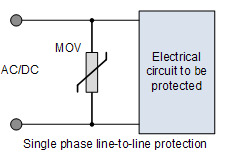2. The following circuit is similar to the previous one except that it provides line-to-ground protection.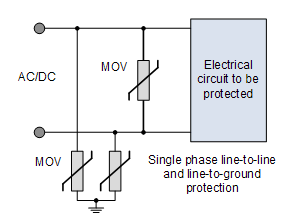3. In electronic circuits, the devices are very sensitive to changes in voltage. Hence varistor is used. The following circuit is to show a typical varistor protecting a transistor.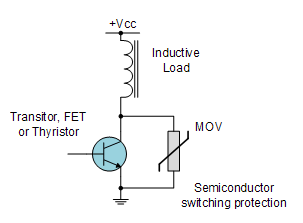4. To provide surge protection to AC or DC motors.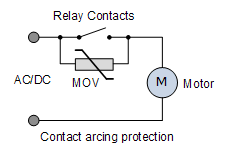### Limitations

When a varistor is used in transient voltage surge suppressor, it may not provide power protection to the device. This is due to the presence of a varistor in this situation will cause problems to equipment and the device itself.

Varistor cannot provide protection from the following:

1. Current surges during device start up
2. Current from short circuit.
3. From voltage sags or brownouts.